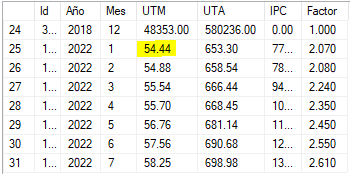# Decimal value gets rounded when entering DB

I’m trying to upload a decimal value into a database.
I get the values ​​from a table and assign them as String

in the output the values ​​are printed well

values ​​arrive in query

“IF EXISTS (Select * From UTM_UTA_IPC Where Año = 2022 And Mes = “+outMes+” ) UPDATE UTM_UTA_IPC Set UTM =”+outUTM+“, UTA = “+outUTA+”, IPC = “+outIPC+” Where Año = 2022 And Mes =”+outMes+"
ELSE
INSERT INTO UTM_UTA_IPC (Año, Mes, UTM, UTA,IPC, Factor ) VALUES ( 2022, “+outMes+”, “+outUTM+”, “+outUTA+”, “+outIPC+”, “+outFactor+”)"

when the values ​​reach the DB they change, they are roundedexample:
correct numberoutput in DBThis is because you declared at the creation of your table in the database the UTM column to be something like

``````UTM DECIMAL(10,2)
``````

You should change the column to

``````UTM DECIMAL(10,3)
``````

Hi @Amanda_Hatte
I think constraints are set for Column in Database